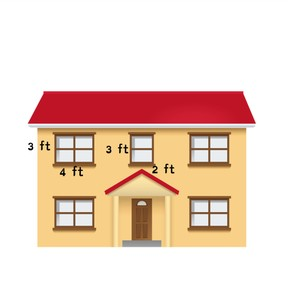Calculating area

# Calculating area

I can calculate the area of a square or rectangle.8,000 schools use Gynzy92,000 teachers use Gynzy1,600,000 students use Gynzy

## General

Students will learn the meaning of area. They will apply the formulas for the area of a square and rectangle to calculate the area in square units.

## Standards

CCSS.Math.Content.4.MD.A.3

## Learning objective

Students will be able to calculate the area of a square or rectangle.

## Introduction

Students complete several rounds of practice with basic multiplication facts. They also review finding a missing factor. They learn that these skills are needed to calculate area.

## Instruction

Students learn the meaning of area as the size a surface takes up. They review that area is always measured in square units such as feet or meters. Next, students learn the two formulas for area as they relate to a square ( a = s x s) and rectangle (a = l x w). Practice is provided finding the area of various shapes. Moving on, the concept of a missing side is introduced. The area is given with the measurement of either the width or the length. Students think about the missing factor to find the measurement of the missing side. For further drill, students complete charts that require them to find the area as well as the missing side. Students then solve two more exercises that require finding area. For one problem, the numbers used are larger, so anon screen calculator is supplied for them to use.

## Quiz

Students respond to ten multiple-choice and true/false questions.

## Closing

Students will find the area of one square on a checkerboard, then find the area of the entire checkerboard. They are allowed to use a calculator for this. Following this, there is a checkerboard set up for a game as an optional activity.

### The online teaching platform for interactive whiteboards and displays in schools

• Save time building lessons

• Manage the classroom more efficiently

• Increase student engagement